# JP4730073B2 - Permanent magnet synchronous motor vector controller, inverter module, and permanent magnet synchronous motor motor constant display system - Google Patents

Permanent magnet synchronous motor vector controller, inverter module, and permanent magnet synchronous motor motor constant display system Download PDF

## Info

Publication number
JP4730073B2
JP4730073B2 JP2005348614A JP2005348614A JP4730073B2 JP 4730073 B2 JP4730073 B2 JP 4730073B2 JP 2005348614 A JP2005348614 A JP 2005348614A JP 2005348614 A JP2005348614 A JP 2005348614A JP 4730073 B2 JP4730073 B2 JP 4730073B2
Authority
JP
Japan
Prior art keywords
value
motor
axis
permanent magnet
magnet synchronous
Prior art date
Legal status (The legal status is an assumption and is not a legal conclusion. Google has not performed a legal analysis and makes no representation as to the accuracy of the status listed.)
Expired - Fee Related
Application number
JP2005348614A
Other languages
Japanese (ja)
Other versions
JP2007159212A (en
Inventor

Original Assignee

Priority date (The priority date is an assumption and is not a legal conclusion. Google has not performed a legal analysis and makes no representation as to the accuracy of the date listed.)
Filing date
Publication date
Application filed by 株式会社日立製作所 filed Critical 株式会社日立製作所
Priority to JP2005348614A priority Critical patent/JP4730073B2/en
Publication of JP2007159212A publication Critical patent/JP2007159212A/en
Application granted granted Critical
Publication of JP4730073B2 publication Critical patent/JP4730073B2/en
Expired - Fee Related legal-status Critical Current
Anticipated expiration legal-status Critical

• 230000001360 synchronised Effects 0.000 title claims description 47
• 244000171263 Ribes grossularia Species 0.000 claims description 154
• 238000004364 calculation methods Methods 0.000 claims description 118
• 238000006243 chemical reactions Methods 0.000 description 7
• 238000010586 diagrams Methods 0.000 description 6
• 230000000694 effects Effects 0.000 description 3
• 239000000758 substrates Substances 0.000 description 3
• 230000007274 generation of a signal involved in cell-cell signaling Effects 0.000 description 2
• 238000000034 methods Methods 0.000 description 2
• 238000005516 engineering processes Methods 0.000 description 1
• 230000004907 flux Effects 0.000 description 1
• 238000004519 manufacturing process Methods 0.000 description 1

## Images

•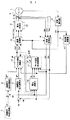•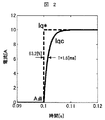•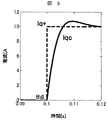•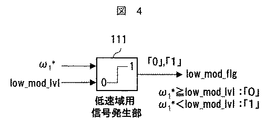•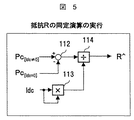•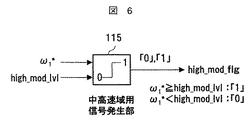•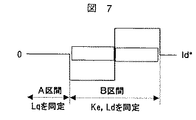•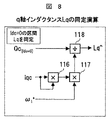•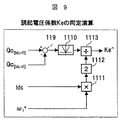•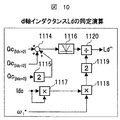•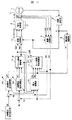•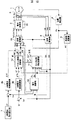•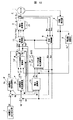•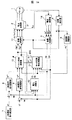•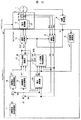## Classifications

• HELECTRICITY
• H02GENERATION; CONVERSION OR DISTRIBUTION OF ELECTRIC POWER
• H02PCONTROL OR REGULATION OF ELECTRIC MOTORS, ELECTRIC GENERATORS OR DYNAMO-ELECTRIC CONVERTERS; CONTROLLING TRANSFORMERS, REACTORS OR CHOKE COILS
• H02P21/00Arrangements or methods for the control of electric machines by vector control, e.g. by control of field orientation
• H02P21/14Estimation or adaptation of machine parameters, e.g. flux, current or voltage
• H02P21/16Estimation of constants, e.g. the rotor time constant

## Description

The present invention relates to a vector control system for a permanent magnet synchronous motor.

As a motor constant identification technique in the permanent magnet synchronous motor vector control system, as described in JP-A-2001-145399, a voltage detector is attached to the power converter, and the detected voltage values of the d-axis and the q-axis are obtained. A technique for calculating and identifying a motor constant from the following three steps is described.

(1) The induced voltage coefficient of the motor is identified from the deviation between the voltage command value of the q-axis (torque) component and the detected voltage value in vector control.
(2) The motor resistance value is identified from the deviation between the voltage command value of the d-axis (magnetic flux) component and the detected voltage value.
(3) When the resistance value of the motor identified in (2) above exceeds the limit value, the operation is switched from the voltage deviation of the d-axis component to the operation for identifying the q-axis inductance.

JP 2001-145399 A

In the method described in Japanese Patent Laid-Open No. 2001-145399, a dedicated voltage detector is required for the power converter, and the “motor constant identification accuracy” deteriorates due to a voltage offset or the like, leading to deterioration of control characteristics. Is concerned.

An object of the present invention is to provide a “permanent magnet motive motor vector control device” that can realize “highly accurate and highly responsive torque control” without newly adding a detector or the like.

As one means for solving the above-mentioned problem, the present invention controls the output voltage value of the power converter by the d-axis and q-axis current command values, current detection values, frequency calculation values, and motor constant setting values. A permanent magnet synchronous motor vector control device for identifying the motor constant using an active power or reactive power obtained from an output voltage value and a current detection value of the power converter, a frequency calculation value, and a current detection value; In the low speed range, there is a section in which the d-axis current command value is set to at least two stages of “positive” or “negative” and “zero”, and the d-axis current command value is set to “positive” or “negative”. The absolute value of the active power in the “zero” section is subtracted from the absolute value of the active power in the “0” section, and the subtraction value is a value obtained by squaring the “positive” or “negative” d-axis current detection value. By performing a division operation, the mode And identifying the resistance value.

As another means for solving the above problem, the present invention determines the output voltage value of the power converter based on the d-axis and q-axis current command values, current detection values, frequency calculation values, and motor constant setting values. Vector control of the permanent magnet synchronous motor that controls and identifies the motor constant using the active power or reactive power obtained from the output voltage value and current detection value of the power converter, the frequency calculation value and the current detection value In the medium and high speed range, the d-axis current command value is set to “zero”, the absolute value of the reactive power is the product of the frequency calculation value and the value obtained by squaring the q-axis current detection value, A q-axis inductance value of the motor is identified by performing a division operation.

ADVANTAGE OF THE INVENTION According to this invention, the vector control apparatus of the permanent magnet synchronous motor which implement | achieves highly accurate and highly responsive torque control can be provided.

The present invention relates to a vector control method of a permanent magnet synchronous motor. The technology relates to high-precision, high-response torque control.

Hereinafter, embodiments of the present invention will be described in detail with reference to the drawings.

[First embodiment]
FIG. 1 shows a configuration example of a vector control apparatus for a permanent magnet synchronous motor according to an embodiment of the present invention. 1 is a permanent magnet synchronous motor, 2 is a power converter that outputs a voltage proportional to the voltage command values Vu * , Vv * , and Vw * of a three-phase AC, 21 is a DC power source, 3 is a three-phase AC current Iu, Iv, A current detector that can detect Iw, 4 is a position detector that can detect the position θ of the motor, 5 is a frequency calculation unit that calculates a frequency calculation value ω 1 * from the position detection value θc, and 6 is a current detection value of a three-phase alternating current. A coordinate conversion unit that outputs current detection values Idc and Iqc of the d-axis and q-axis from Iuc, Ivc, Iwc and the position detection value θc, and 7 is a d-axis current command setting for setting the first d-axis current command value Id * , 8 is a d-axis current command calculation unit that outputs an intermediate second current command value Id ** in accordance with the deviation between the first d-axis current command value Id * and the detected d-axis current value Idc, 9 Is the deviation between the first q-axis current command value Iq * and the q-axis current detection value Iqc given from the host , A q-axis current command calculation unit 10 for outputting an intermediate second q-axis current command value Iq ** , and 10 is a voltage command value Vdc * , Vqc * and a current detection value Idc,
A power calculation unit that calculates the reactive power and active power of the permanent magnet synchronous motor 1 from Iqc and outputs the reactive power calculation value Pc and the active power calculation value Qc, 11 is the reactive power Pc, the active power Qc, and the current detection value Idc , Iqc, motor constant identification calculation unit for calculating the resistance identification value R ^, d-axis and q-axis inductance identification values Ld ^, Lq ^, and induced voltage coefficient identification value Ke ^ of the permanent magnet synchronous motor 1; Reference numeral 12 denotes intermediate second d-axis and q-axis current command values Id ** , Iq ** , frequency calculation value ω 1 *, and motor constant setting values (R * , Ld * , Lq * , Ke * ). Or a vector control arithmetic unit that outputs voltage command values Vdc * and Vqc * using (R ^, Ld ^, Lq ^, Ke ^) that are output values of the motor constant identification calculating unit 11, and 13 is a voltage command Values Vdc * , Vqc * and position detection value θ It is a coordinate conversion unit that outputs three-phase AC voltage command values Vu * , Vv * , Vw * from c.

First, a basic voltage and phase control method in the permanent magnet synchronous motor vector control device will be described.

The basic operation of the voltage control is based on vector control using the first current command values Id * and Iq * and the current detection values Idc and Iqc given from the host in the d-axis and q-axis current command calculation units 8 and 9. Second intermediate current command values Id ** and Iq ** used for calculation are calculated.

The vector control calculation unit 12 uses the second current command values Id ** , Iq ** , the frequency command value ω 1 *, and the motor constant setting value, and the voltage command values Vd ** , Vq ** is calculated and the voltage command values Vu * , Vv * , Vv * of the power converter are controlled.

Where R: resistance value, Ld: d-axis inductance value, Lq: q-axis inductance value,
Ke: induced voltage coefficient, *: set value.

On the other hand, for the basic operation of phase control, the position detector 4 obtains the position detection value θc of the permanent magnet synchronous motor 1. The frequency calculation unit 5 calculates a frequency calculation value ω 1 * shown in the equation (2) from the position detection value θc.

Further, the phase of the permanent magnet synchronous motor 1 is controlled by setting the reference phase of the coordinate conversion units 6 and 13 to the position detection value θc.

The above is the basic operation of voltage control and phase control in the vector controller of the permanent magnet synchronous motor.

Next, control characteristics in a case where the motor constant identification calculation unit 11 which is a feature of the present invention is not provided (setting values of motor constants are R * , Ld * , Lq * , Ke * ) will be described.

FIG. 2 and FIG. 3 show current step response characteristics when there is a motor constant setting error in the control device of FIG.

FIG. 2 shows a case where the d-axis and q-axis inductance values Ld and Lq of the permanent magnet synchronous motor 1 coincide with the set values Ld * and Lq * set in the vector control calculation unit 12 (Ld * = Ld, Lq * = Lq).

When the motor is operating at a constant speed, if the q-axis current command value Iq * is step-changed as indicated by the broken line at point A at time 0.1 [s], the q-axis current detection value Iqc is a response. It can be seen that at time 1.6 [ms], it follows quickly without overshoot.

On the other hand, in FIG. 3, which is a characteristic when the inductance values Ld and Lq of the motor do not match the set values Ld * and Lq * (Ld * = 0.5 × Ld, Lq * = 0.5 × Lq), It can be seen that the follow-up of the q-axis current detection value Iqc is slower than the command value Iq * , and overshoot is also occurring.

In other words, if there is a setting error of the motor constant, the follow-up characteristic to the current command value is deteriorated, which eventually causes a problem of torque response and torque accuracy.

Hereinafter, the “principle of motor constant identification” using the active power and reactive power of the permanent magnet synchronous motor 1 will be described.

The applied voltages Vd and Vq on the d-axis and q-axis of the permanent magnet synchronous motor 1 are expressed by using motor currents Id and Iq and motor constants.

First, “the identification principle of the resistance R” focusing on the active power P will be described.

The effective power P of the permanent magnet synchronous motor 1 can be expressed by the number (4).

Here, from the numbers (3) and (4),

Also, assuming that the active power calculated on the control side is Pc,

Assume that the motor axis and the control axis match (active power P and active power calculation value Pc match), and in equation (5), motor currents Id and Iq are detected current values Idc and Iqc and motor frequency ω 1 is set. If you put the frequency calculation value ω 1 * ,

In Equation (7), if the frequency calculation value ω 1 * is near “zero”,

Here, when a section in which the first d-axis current command value Id * is set between two stages of “zero” and “positive” or “negative” is provided, the active power generated in each is expressed as Pc [Idc = 0 ] , Pc [Idc ≠ 0] and the calculation of the number (9)

From the equation (10), the identification value of the resistor R can be calculated.

Next, the “identification principle of the d-axis and q-axis inductances Ld and Lq and the induced voltage coefficient Ke” focusing on the reactive power Q will be described.

The reactive power Q of the permanent magnet synchronous motor 1 can be expressed by the number (11).

Here, from the numbers (3) and (11),

Further, when the reactive power calculated on the control side is Qc,

Here, assuming that the motor axis and the control axis coincide with each other, and in Equation (12), if the motor currents Id and Iq are the current detection values Idc and Iqc, and the motor frequency ω 1 is the frequency calculation value ω 1 * ,

In equation (14), it can be seen that the reactive power calculation value Qc includes the d- and q-axis inductances Ld and Lq of the motor constant and the induced voltage coefficient Ke.

First, “identification principle of q-axis inductance Lq” will be described.

In the d-axis current command setting unit 7 in FIG. 1, the d-axis current command value Id * is set to “zero”. At this time, the reactive power calculation value Qc calculated by the power calculation unit 10 is set to Qc [Idc = 0]. Then,

The identification value Lq ^ of the q-axis inductance Lq can be calculated from the equation (16).

Second, the “identification principle of the induced voltage coefficient Ke” will be described.

When the first d-axis current command value Id * is set with a section set between “positive” and “negative”, the reactive power calculation value Qc [Idc> 0] calculated in each section is set . , Qc [Idc <0] is used to calculate the number (17),

The identification value Ke ^ of the induced voltage coefficient Ke can be calculated from the number (18).

Third, the “identification principle of the d-axis inductance Ld” will be described. Next, when the calculation of the number (19) is performed using the reactive power calculation values Qc [Idc> 0] and Qc [Idc <0] ,

Here, from the number (15) and the number (19),

The identification value Ld ^ of the d-axis inductance Ld can be calculated from the number (21).

This completes the explanation of the “motor constant identification principle”.

Here, the “configuration of the motor constant identification calculation unit 11” which is characteristic of the present invention will be described with reference to FIGS. 1, 4, and 5.

First, “resistance R identification calculation” executed in the “low speed region”, which is a feature of the present invention, will be described.

In FIG. 4, the frequency calculation value ω 1 * is input to the signal generation unit 111 that determines the “low speed range” section. The signal generator 111 compares ω 1 * with the low speed region detection level (low_mod_lvl), and creates a determination flag (low_mod_flg) based on the relationship of the number (22).

When the determination flag is “1”, the low speed region is determined and the resistance R is identified.

Here, the low speed region detection level is a value of several percent or less of the motor rated rotational speed.

In the d-axis current command setting unit 7 in FIG. 1, the d-axis current command value Id * is set to “zero” and “positive”.
(Or “negative”).

Next, specific contents of the “resistance R identification calculation” will be described with reference to FIG.

The subtractor 112 includes an active power calculation value Pc [Idc ≠ 0] that is an output signal of the power calculation unit 10 when the d-axis current command value Id * is set to “positive”, and a d-axis current command value Id *. Calculated Pc [Idc = 0], which is an output signal of the power calculation unit 10 when is set to “zero”, is input to the output signal of the d-axis current detection value Idc which is an output signal of the multiplication unit 113. Together with the squared value, it is input to the division unit 114. The output signal of the dividing unit 114 becomes the identification value R ^ of the resistor R.

Second, “identification calculation of d-axis and q-axis inductances Ld, Lq and induced voltage coefficient Ke” executed in the “medium / high speed region” will be described with reference to FIGS.

The frequency calculation value ω 1 * is input to the signal generation unit 115 that determines the “medium / high speed range” section. The signal generator 115 compares ω 1 * and the medium / high speed region detection level (high_mod_lvl), and creates a determination flag (high_mod_flg) based on the relationship of the number (23).

When the determination flag is “1”, it is determined as the “high speed region” section, and the identification calculation of “inductance value and induced voltage coefficient” is performed. Here, the high speed region detection level is a value of about 10% or more of the motor rated rotation speed.

FIG. 7 shows the relationship between the set pattern of the first d-axis current command value Id * and three (Ld, Lq, Ke) identification intervals.

In a section where Idc is “zero” (A section), the q-axis inductance Lq is identified. Next, in a section where Idc is “positive” and “negative” (B section), the d-axis inductance value Ld and the induced voltage are identified. The coefficient Ke is identified.

This will be described with reference to FIGS.

First, “identification calculation of q-axis inductance Lq” will be described with reference to FIG.

Multiplier 116 receives d-axis current detection value Idc and outputs a value obtained by squaring Idc. The multiplier 117 receives the frequency calculation value ω 1 * and the output signal of the multiplier 116.

Qc [Idc = 0] , which is an output signal of the power calculation unit 10 when the d-axis current command value Id * is set to “zero”, and an output signal of the multiplication unit 117 are input to the division unit 118.

The output signal of the dividing unit 118 becomes the identification value Lq ^ of the q-axis inductance Lq.

Secondly, the “identification calculation of the induced voltage coefficient Ke” will be described with reference to FIG.

The subtraction unit 119 displays the reactive power Qc [Idc> 0] that is the output of the power calculation unit 10 when the d-axis current command value Id * is set to “positive” and the d-axis current command value Id * as “negative”. Qc [Idc <0], which is an output of the power calculation unit 10 when set to “”, is input to the absolute value calculation unit 1110.

Further, the d-axis current detection value Idc and the frequency calculation value ω 1 * are input to the multiplication unit 1111, and the output signal is multiplied by “2” which is a constant value 1112 to output the output signal of the absolute value calculation unit 1110. At the same time, it is input to the division unit 1113.

The output signal of the dividing unit 1113 becomes the identification value Ke ^ of the induced voltage coefficient Ke.

Thirdly, “identification calculation of d-axis inductance Ld” will be described with reference to FIG. The subtraction unit 1114 displays the reactive power Pc [Idc> 0] , which is the output of the power calculation unit 10 when the d-axis current command value Id * is set to “positive”, and the d-axis current command value Id * as “negative”. Pc [Idc <0] , which is the output of the power calculation unit 10 when set to “,” and Pc [Idc = Id, which is the output of the power calculation unit 10 when the d-axis current command value Id * is set to “zero”.  is multiplied by a constant value 1115 “2”. The output signal is input to the absolute value calculation unit 1116.

The multiplication unit 1117 receives the d-axis current detection value Idc and outputs a value obtained by squaring Idc. The multiplier 1118 receives the frequency calculation value ω 1 * and the output signal of the multiplier 1117. The output signal is multiplied by a constant value “1119” “2” and input to the division unit 1120 together with the output signal of the absolute value calculation unit 1116.

The output signal of the division unit 1120 becomes the identification value Ld ^ of the d-axis inductance Ld.

Here, the vector control calculation unit 12 calculates the voltage command values Vdc * and Vqc * shown in Equation (24) using the identification value of the permanent magnet synchronous motor 1 obtained by the calculation, and the voltage command of the power converter. By controlling the values Vu * , Vv * , and Vv * , it is possible to provide a permanent magnet synchronous motor vector control device that realizes high-accuracy and high-response torque control immediately before or during actual operation.

[Second embodiment]
In the first embodiment, the motor constant of the vector control calculation unit 12 is set using the output values (R ^, Ld ^, Lq ^, Ke ^) of the motor constant identification calculation unit 11, 11 output values can also be applied to the correction of the control gains of the second d-axis and q-axis current command calculation units 8a and 9a.

FIG. 11 shows this embodiment. In the figure, 1-7, 10-13 and 21 are the same as those in FIG.

As shown in Equation (25), if the identified motor constants (R ^, Ld ^, Lq ^) are used, the control gains (Kp_d, Ki_d, Kp_q, Ki_q) of the current command calculation units 8a, 9a are corrected. A torque control system with high accuracy and high response can be realized.

Here, Kp_d: proportional gain for second d-axis current command calculation, Ki_d: integral gain, Kp_q: proportional gain for second q-axis current command calculation, Ki_q: integral gain, ω c _acr: current control response Angular frequency [rad / s].

[Third embodiment]
FIG. 12 shows another embodiment of the present invention. The present embodiment is a vector control device for a permanent magnet same-machine motor that corrects the output values Vdc * and Vqc * of the vector control calculation with the deviation between the current command value and the current detection value given from the host.

In the figure, 1-7, 10, 11, 13, 21 are the same as those in FIG. 8b is a d-axis current control calculation unit that calculates the d-axis correction voltage ΔVd so that the d-axis current command value Id * and the d-axis current detection value Idc match, and 9b is the q-axis current command value Iq * and the q-axis current. A q-axis current control calculation unit 12 ′ that calculates a q-axis correction voltage ΔVq so that the detection value Iqc matches, d ′ and q-axis current command values Id * and Iq * , a frequency calculation value ω 1 *, and a motor This is a vector control calculation unit that outputs voltage command values Vdc * and Vqc * using (R ^, Ld ^, Lq ^, Ke ^) that are output values of the constant identification calculation unit 11.

The difference from FIG. 1 shown in the previous embodiment is that, in the d-axis and q-axis current control calculation units 8b and 9b, the current command values Id * and Iq * given from the higher order are detected current values Idc and Iqc. The voltage correction values ΔVd and ΔVq are calculated so as to coincide.

In addition, the vector control calculation unit 12 ′ uses the current command values Id * and Iq * , the frequency command value ω 1 * and the motor constant identification value, and the voltage command reference values Vdc * and Vqc * shown in the equation (26) . And

As shown by the equation (27), the voltage command values Vdc * ′ and Vqc * ′ of the power converter are calculated.

It is obvious that such a vector control operation operates in the same manner as in the above embodiment, and the same effect can be obtained.

[Fourth embodiment]
In the third embodiment, the motor constants of the vector control arithmetic unit 12 ′ are set using the output values (R ^, Ld ^, Lq ^, Ke ^) of the motor constant identification arithmetic unit 11. , 11 can be used to correct the control gains of the d-axis and q-axis current command calculation units 8c and 9c.

FIG. 13 shows this embodiment. In the figure, 1 to 7, 10, 11, 12 ', 13, 21 are the same as those in FIG.

As shown in equation (28), using the identified motor constants (R ^, Ld ^, Lq ^), the control gains (Kp_d1, Ki_d1, Kp_q1, Ki_) of the current command calculation units 8c, 9c are used.
If q1) is corrected, a highly accurate and highly responsive torque control system can be realized.

Here, Kp_d1: proportional gain for d-axis current control calculation, Ki_d1: integral gain,
Kp_q1: proportional gain for q-axis current control calculation, Ki_q1: integral gain, ω c — acr: current control response angular frequency [rad / s].

[Fifth embodiment]
In the first embodiment, the three-phase alternating currents Iu to Iw are detected by the expensive current detector 3, but the present invention can also be applied to a control device that performs inexpensive current detection.

FIG. 14 shows this embodiment. In the figure, 1, 2, 4 to 13, 21 are the same as those in FIG. 14 from the DC current I DC flowing through the input bus of the power converter, a current reproduction unit which estimates alternating currents Iu 3 phase flowing to the permanent magnet synchronous motor 1, Iv, and Iw.

Using the estimated current values Iu ^, Iv ^, Iw ^, the coordinate conversion unit 6 calculates current detection values Idc, Iqc for the d-axis and the q-axis. It is obvious that such a current sensorless system operates in the same manner as the above-described embodiment and is also inexpensive, while being inexpensive.

Also, when applied to the second, third, and fourth embodiments, it is obvious that the operation is the same as the above-described embodiment and the same effect can be obtained while being inexpensive.

[Sixth embodiment]
An example in which the present invention is applied to a module will be described with reference to FIG. This example shows an embodiment of the sixth example.

Here, a frequency calculation unit 5, a coordinate conversion unit 6, a d-axis current command setting unit 7, a d-axis current command calculation unit 8, a q-axis current command calculation unit 9, a power calculation unit 10, a motor constant identification calculation unit 11, a vector The control calculation unit 12 and the coordinate conversion unit 13 are configured using a one-chip microcomputer.

Further, the one-chip microcomputer and the power converter are housed in one module configured on the same substrate. The module here means “standardized structural unit” and is composed of separable hardware / software components. In addition, although it is preferable that it is comprised on the same board | substrate on manufacture, it is not limited to the same board | substrate. From this, it may be configured on a plurality of circuit boards built in the same housing. In other embodiments, the same configuration can be adopted.

[Seventh embodiment]
In the embodiments so far, the motor constant is calculated by the motor constant identification calculation unit 11 and the motor constant of the vector control calculation unit is identified. However, the motor constant is calculated by the motor constant identification calculation unit 11 and displayed. It is also possible to do.

Thereby, the change of the characteristic of a permanent magnet motor can be visualized. Further, the degree of deterioration of the permanent magnet motor can be recognized by visualization. And when it deteriorates to some extent, it also has the effect of knowing when to replace the motor.

The block diagram of the vector control apparatus of the permanent magnet motor which shows one Example of this invention. Current control characteristics when the motor inductance value and the inductance setting value set in the vector calculation match (Ld * = Ld, Lq * = Lq). Current control characteristics when the motor inductance value does not match the inductance setting value set in the vector calculation (Ld * = 0.5 × Ld, Lq * = 0.5 × Lq). Explanatory drawing of the motor constant identification calculating part 11 in the control apparatus of FIG. Explanatory drawing of the motor constant identification calculating part 11 in the control apparatus of FIG. Explanatory drawing of the motor constant identification calculating part 11 in the control apparatus of FIG. Explanatory drawing of the motor constant identification calculating part 11 in the control apparatus of FIG. Explanatory drawing of the motor constant identification calculating part 11 in the control apparatus of FIG. Explanatory drawing of the motor constant identification calculating part 11 in the control apparatus of FIG. Explanatory drawing of the motor constant identification calculating part 11 in the control apparatus of FIG. The block diagram of the vector control apparatus of the permanent magnet synchronous motor which shows the other Example of this invention. The block diagram of the vector control apparatus of the permanent magnet synchronous motor which shows the other Example of this invention. The block diagram of the vector control apparatus of the permanent magnet synchronous motor which shows the other Example of this invention. The block diagram of the vector control apparatus of the permanent magnet synchronous motor which shows the other Example of this invention. An example of the block diagram which shows embodiment of this invention.

Explanation of symbols

DESCRIPTION OF SYMBOLS 1 ... Permanent magnet synchronous motor, 2 ... Inverter, 3 ... Current detector, 4 ... Position detector, 5 ... Frequency calculating part, 6, 13 ... Coordinate conversion part, 7 ... d-axis current command setting part, 8, 8a ... d-axis current command calculation unit, 8b, 8c ... d-axis current control calculation unit, 9, 9a ... q-axis current command calculation unit, 9b, 9c ... q-axis current control calculation unit, 10 ... power calculation unit, 11 ... motor constant Identification calculation unit, 12, 12 '... vector control calculation unit, 14 ... current reproduction unit, R ^ ... identification value of resistance R, Ke ^ ... identification value of induced voltage coefficient Ke, Ld ^ ... identification value of d-axis inductance Ld , Lq ^ ... identified value of q-axis inductance Lq, Id * ... first d-axis current command value, Id ** ... second d-axis current command value, Iq * ... first q-axis current command value, Iq ** … second q-axis current command value, Vd ** … d-axis voltage command value, Vq ** … q-axis voltage command value , Ω 1 * ... frequency calculation value, I DC ... input DC bus current detection value.

## Claims (12)

1. The output voltage value of the power converter is controlled by the d-axis and q-axis current command values, current detection values, frequency calculation values, and motor constant setting values. A permanent magnet synchronous motor vector control device for identifying the motor constant using the active power or reactive power obtained from the frequency calculation value and current detection value;
In the low speed range, a section is provided in which the d-axis current command value is set to at least two stages of “positive” or “negative” and “zero”, and the d-axis current command value is “positive” or “negative”. The absolute value of the active power in the “zero” section is subtracted from the absolute value of the active power at, and the subtraction value is divided by the square of the “positive” or “negative” d-axis current detection value. By doing so, the resistance value of the said motor is identified, The vector control apparatus of the permanent-magnet synchronous motor characterized by the above-mentioned.
2. The output voltage value of the power converter is controlled by the d-axis and q-axis current command values, current detection values, frequency calculation values, and motor constant setting values. A permanent magnet synchronous motor vector control device for identifying the motor constant using the active power or reactive power obtained from the frequency calculation value and current detection value;
In the middle / high speed range, the d-axis current command value is set to “zero”, and the absolute value of the reactive power is divided by the product of the frequency calculation value and the q-axis current detection value squared. A vector controller for a permanent magnet synchronous motor, characterized by identifying a q-axis inductance value of the motor.
3. The output voltage value of the power converter is controlled by the d-axis and q-axis current command values, current detection values, frequency calculation values, and motor constant setting values. A permanent magnet synchronous motor vector control device for identifying the motor constant using the active power or reactive power obtained from the frequency calculation value and current detection value;
In the middle / high speed range, there is a section in which the d-axis current command value is set to at least two levels of “positive” and “negative”, and the d-axis current command value is determined from the absolute value of reactive power in the “positive” section. , By subtracting the absolute value of the reactive power in the “negative” section and dividing the subtracted value by the product of the frequency calculation value and the d-axis current detection value to identify the induced voltage coefficient value of the motor A vector control device for a permanent magnet synchronous motor.
4. The output voltage value of the power converter is controlled by the d-axis and q-axis current command values, current detection values, frequency calculation values, and motor constant setting values. A permanent magnet synchronous motor vector control device for identifying the motor constant using the active power or reactive power obtained from the frequency calculation value and current detection value;
In the middle / high speed range, there is a section where the d-axis current command value is set to at least three levels of “positive”, “negative” and “zero”.
The d-axis current command value adds the absolute value of reactive power in the “positive” section, the absolute value of reactive power in the “negative” section, and the equivalent of twice the absolute value of reactive power in the “zero” section. The addition value is equivalent to twice the multiplication value of the frequency operation value and the square value of the d-axis current detection value, and the d-axis inductance value of the motor is identified by performing a division operation. Vector controller for permanent magnet synchronous motor.
5. In any of claims 1 to 4 ,
The output voltage value of the power converter is calculated from the second current command value calculated from the d-axis and q-axis current command values and the current detection value, the motor constant, and the frequency calculation value. Vector controller for synchronous motor.
6. In any of claims 1 to 4 ,
The output voltage value of the power converter includes the output value of the vector control calculation unit calculated using the first d-axis and q-axis current command values, the frequency calculation value, and the motor constant, and the motor current detection value. A vector control device for a permanent magnet synchronous motor, wherein the vector control device is an added value to an output value of a current control arithmetic unit controlled to follow the first current command value.
7. In any of claims 1 to 4 ,
A vector control device for a permanent magnet synchronous motor, wherein a set value of a motor constant used for calculation of an output voltage value of a power converter is corrected using a motor constant obtained by identification calculation.
8. In claim 5 ,
A vector control apparatus for a permanent magnet synchronous motor, wherein a control gain for calculating a second current command value is corrected using a motor constant obtained by identification calculation.
9. In claim 6 ,
A vector control device for a permanent magnet synchronous motor, wherein a control gain for calculating an output value of a current control calculation unit is corrected using a motor constant obtained by identification calculation.
10. In any of claims 1 to 4 ,
The vector detection device for a permanent magnet synchronous motor, wherein the current detection value is a current that reproduces a motor current from an input bus direct current detection value of a power converter.
11. In the inverter module which has the vector controller of the permanent magnet synchronous motor according to any one of claims 1 to 10, and the power converter,
Using the active power or reactive power obtained from the output voltage value and current detection value of the power converter, the frequency calculation value and the current detection value,
An inverter module for identifying the motor constant.
12. In the permanent magnet synchronous motor motor constant display system comprising the permanent magnet synchronous motor vector control device and the display device according to any one of claims 1 to 10 ,
A motor constant calculation unit for obtaining a motor constant using the active power or reactive power obtained from the output voltage value and current detection value of the power converter, the frequency calculation value and the current detection value; A permanent magnet synchronous motor motor constant display system characterized by displaying on a display device.
JP2005348614A 2005-12-02 2005-12-02 Permanent magnet synchronous motor vector controller, inverter module, and permanent magnet synchronous motor motor constant display system Expired - Fee Related JP4730073B2 (en)

## Priority Applications (1)

Application Number Priority Date Filing Date Title
JP2005348614A JP4730073B2 (en) 2005-12-02 2005-12-02 Permanent magnet synchronous motor vector controller, inverter module, and permanent magnet synchronous motor motor constant display system

## Applications Claiming Priority (3)

Application Number Priority Date Filing Date Title
JP2005348614A JP4730073B2 (en) 2005-12-02 2005-12-02 Permanent magnet synchronous motor vector controller, inverter module, and permanent magnet synchronous motor motor constant display system
CNB2006101636185A CN100530935C (en) 2005-12-02 2006-12-01 Vector controller for a permanent magnet synchronous motor
US11/606,976 US7521887B2 (en) 2005-12-02 2006-12-01 Vector controller for a permanent magnet synchronous motor, inverter module, and permanent magnet synchronous motor constant display system

## Publications (2)

Publication Number Publication Date
JP2007159212A JP2007159212A (en) 2007-06-21
JP4730073B2 true JP4730073B2 (en) 2011-07-20

# Family

## Family Applications (1)

Application Number Title Priority Date Filing Date
JP2005348614A Expired - Fee Related JP4730073B2 (en) 2005-12-02 2005-12-02 Permanent magnet synchronous motor vector controller, inverter module, and permanent magnet synchronous motor motor constant display system

## Country Status (3)

US (1) US7521887B2 (en)
JP (1) JP4730073B2 (en)
CN (1) CN100530935C (en)

## Families Citing this family (18)

* Cited by examiner, † Cited by third party
Publication number Priority date Publication date Assignee Title
US7772721B2 (en) * 2007-10-03 2010-08-10 Qualcomm Incorporated Method and apparatus for conserving energy stored in bypass capacitors during dynamic power collapse
JP5509538B2 (en) * 2008-05-12 2014-06-04 富士電機株式会社 Control device for permanent magnet type synchronous motor
JP5334524B2 (en) * 2008-10-20 2013-11-06 株式会社日立製作所 Permanent magnet synchronous motor control device and control method thereof
US7804184B2 (en) * 2009-01-23 2010-09-28 General Electric Company System and method for control of a grid connected power generating system
JP2011211815A (en) * 2010-03-30 2011-10-20 Kokusan Denki Co Ltd Controller of permanent magnet motor
JP5413400B2 (en) * 2011-04-20 2014-02-12 株式会社安川電機 AC motor control device
CN103185839B (en) * 2011-12-30 2015-07-08 台达电子企业管理（上海）有限公司 Permanent magnet motor inductance parameter measurement device and permanent magnet motor inductance parameter measurement method
JP5778045B2 (en) * 2012-01-16 2015-09-16 日立アプライアンス株式会社 Synchronous motor drive device, refrigeration apparatus, air conditioner, refrigerator, and synchronous motor drive method using the same
US8848400B2 (en) * 2012-02-15 2014-09-30 General Electric Company System and method for reactive power regulation
WO2014073031A1 (en) * 2012-11-06 2014-05-15 パイオニア株式会社 Motor control device and motor control method
WO2014132395A1 (en) * 2013-02-28 2014-09-04 三菱電機株式会社 Control device for ac rotating machine
KR101493511B1 (en) * 2013-06-26 2015-02-16 포스코에너지 주식회사 An apparatus and a method for controlling an overcurrent of a grid connected inverter according to error of grid voltage
JP6197655B2 (en) * 2014-01-09 2017-09-20 スズキ株式会社 Control device for permanent magnet synchronous motor
JP6261396B2 (en) * 2014-03-13 2018-01-17 日本特殊陶業株式会社 Permanent magnet type synchronous motor vector control device and magnet magnetic flux estimation device
CN103997267B (en) * 2014-04-11 2016-05-11 浙江大学 A kind of series compensation Direct Torque Control of driving winding permanent magnet synchronous motor
JP6514969B2 (en) * 2015-06-24 2019-05-15 日本特殊陶業株式会社 Vector controller for permanent magnet synchronous motor
JP6233428B2 (en) * 2016-02-12 2017-11-22 株式会社安川電機 Motor control device and motor control method
WO2017187533A1 (en) * 2016-04-26 2017-11-02 三菱電機株式会社 Motor drive device, electric cleaner, and hand dryer

## Citations (1)

* Cited by examiner, † Cited by third party
Publication number Priority date Publication date Assignee Title
JP2004297966A (en) * 2003-03-28 2004-10-21 Hitachi Ltd Ac motor controlling device

## Family Cites Families (6)

* Cited by examiner, † Cited by third party
Publication number Priority date Publication date Assignee Title
JP3019653B2 (en) * 1993-03-12 2000-03-13 株式会社日立製作所 Control device for AC motor and method for measuring constants of AC motor
JP3951075B2 (en) * 1998-02-13 2007-08-01 株式会社安川電機 Method and apparatus for controlling motor
JP4225657B2 (en) 1999-11-15 2009-02-18 東洋電機製造株式会社 Control device for permanent magnet type synchronous motor
DE60200431T2 (en) * 2001-03-02 2005-04-07 Matsushita Electric Industrial Co., Ltd., Kadoma Controller for an electric motor
US7102305B2 (en) * 2003-05-22 2006-09-05 Toyoda Koki Kabushiki Kaisha Apparatus and method for controlling motor
CN100417004C (en) * 2003-07-23 2008-09-03 松下电器产业株式会社 Motor controlling equipment and washer and dryer using the same

## Patent Citations (1)

* Cited by examiner, † Cited by third party
Publication number Priority date Publication date Assignee Title
JP2004297966A (en) * 2003-03-28 2004-10-21 Hitachi Ltd Ac motor controlling device

## Also Published As

Publication number Publication date
US20070126391A1 (en) 2007-06-07
US7521887B2 (en) 2009-04-21
CN1976212A (en) 2007-06-06
CN100530935C (en) 2009-08-19
JP2007159212A (en) 2007-06-21

## Similar Documents

Publication Publication Date Title
EP2582036B1 (en) Parameter estimating apparatus for permanent magnet synchronous motor driving system
JP3411878B2 (en) Method for estimating rotor position of synchronous motor, control method without position sensor, and control device
KR100455630B1 (en) Sensorless control method and apparatus of permanent magnet synchronous motor
JP4082444B1 (en) Vector controller for permanent magnet synchronous motor
JP5130716B2 (en) Motor control device and electric power steering device
JP3860031B2 (en) Synchronous motor control device and control method of synchronous motor
EP1748550B1 (en) Synchronous machine controller
US6674262B2 (en) Motor control apparatus and motor control method
US7548038B2 (en) Controller for motor
JP4284355B2 (en) High response control device for permanent magnet motor
US10158305B2 (en) Inverter controller and motor driving system
JP4654217B2 (en) Field weakening control device for permanent magnet motor and electric power steering using the same
US6414462B2 (en) Speed control apparatus for synchronous reluctance motor
JP4357967B2 (en) Control device for synchronous reluctance motor
US6933701B2 (en) Control apparatus for synchronous motor
US9112436B2 (en) System for controlling controlled variable of rotary machine
JP5124483B2 (en) Method and apparatus for driving a synchronous machine
JP5155344B2 (en) Electric motor magnetic pole position estimation device
JP5104239B2 (en) Control device for permanent magnet type synchronous motor
US7230398B2 (en) Brushless motor control apparatus and brushless motor control method
JP4988329B2 (en) Beatless control device for permanent magnet motor
JP5494760B2 (en) Electric motor control device
EP2020743B1 (en) Sensorless controlling apparatus for controlling a brushless motor
JP5130031B2 (en) Position sensorless control device for permanent magnet motor
JP2007110837A (en) Method for estimating magnetic pole position and motor controller

## Legal Events

Date Code Title Description
A621 Written request for application examination

Free format text: JAPANESE INTERMEDIATE CODE: A621

Effective date: 20080311

A977 Report on retrieval

Free format text: JAPANESE INTERMEDIATE CODE: A971007

Effective date: 20101209

A131 Notification of reasons for refusal

Free format text: JAPANESE INTERMEDIATE CODE: A131

Effective date: 20101214

A521 Written amendment

Free format text: JAPANESE INTERMEDIATE CODE: A523

Effective date: 20110207

TRDD Decision of grant or rejection written
A01 Written decision to grant a patent or to grant a registration (utility model)

Free format text: JAPANESE INTERMEDIATE CODE: A01

Effective date: 20110322

A61 First payment of annual fees (during grant procedure)

Free format text: JAPANESE INTERMEDIATE CODE: A61

Effective date: 20110404

R151 Written notification of patent or utility model registration

Ref document number: 4730073

Country of ref document: JP

Free format text: JAPANESE INTERMEDIATE CODE: R151

FPAY Renewal fee payment (event date is renewal date of database)

Free format text: PAYMENT UNTIL: 20140428

Year of fee payment: 3

FPAY Renewal fee payment (event date is renewal date of database)

Free format text: PAYMENT UNTIL: 20140428

Year of fee payment: 3

S111 Request for change of ownership or part of ownership

Free format text: JAPANESE INTERMEDIATE CODE: R313111

R350 Written notification of registration of transfer

Free format text: JAPANESE INTERMEDIATE CODE: R350

LAPS Cancellation because of no payment of annual fees